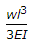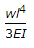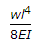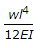# Civil Engineering - Theory of Structures

Exercise : Theory of Structures - Section 1
26.
If E, N, K and 1/m are modulus of elasticity, modulus of rigidity. Bulk modulus and Poisson ratio of the material, the following relationship holds good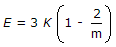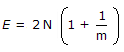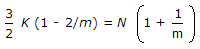all the above.
Explanation:
No answer description is available. Let's discuss.

27.
The ratio of maximum shear stress to average shear stress of a circular beam, is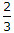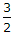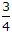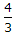Explanation:
No answer description is available. Let's discuss.

28.
A rolled steel joist is simply supported at its ends and carries a uniformly distributed load which causes a maximum deflection of 10 mm and slope at the ends of 0.002 radian. The length of the joist will be,
10 m
12 m
14 m
16 m
18 m
Explanation:
No answer description is available. Let's discuss.

29.
A steel rod 1 metre long having square cross section is pulled under a tensile load of 8 tonnes. The extension in the rod was 1 mm only. If Esteel = 2 x 106 kg/cm2, the side of the rod, is
1 cm
1.5 cm
2 cm
2.5 cm